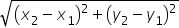Maths-
General
Easy

Question

# Find the distance between (2,-2) and (-6,4)

## The correct answer is: 10 units

### On comparing ordered pair (2,-2),(-6,4) with (x1,y1 ),(x2,y2 ) respectively.We nave x1=2; y1=-1; x2=-6; y2=4Distance between order pair is= 10 units#### With Turito Foundation.#### Get an Expert Advice From Turito.